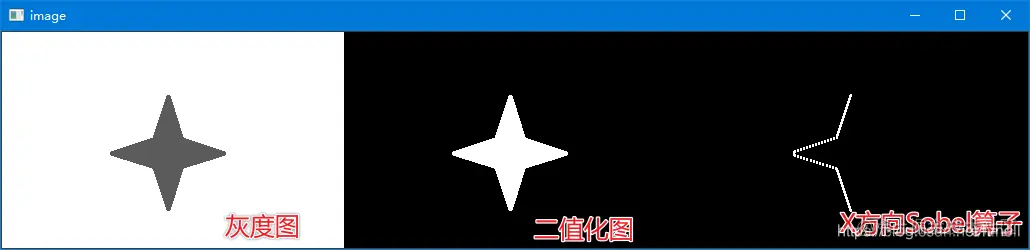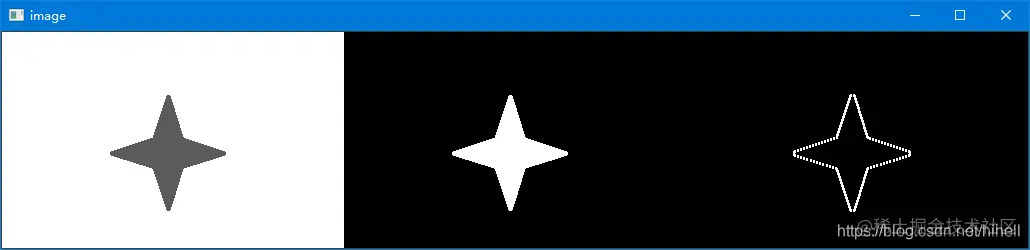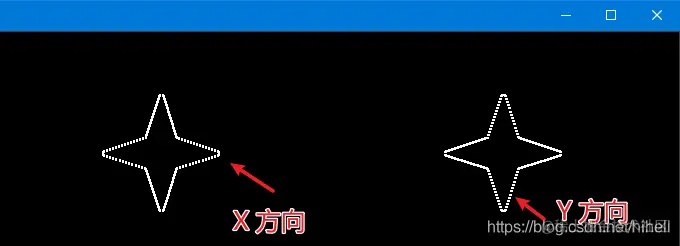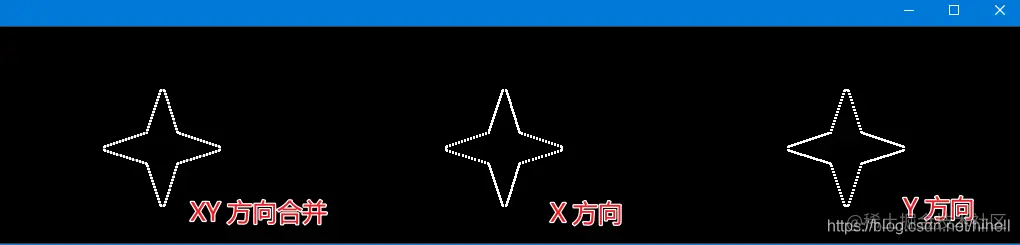# Complex learning of Python opencv Sobel operator, ScHARR operator and Laplacian operator

2022-01-30 07:22:52

Little knowledge , Great challenge ！ This article is participating in 「 A programmer must have a little knowledge 」 Creative activities

This article has participated in  「 Digging force Star Program 」 , Win a creative gift bag , Challenge creation incentive fund .

Python OpenCV 365 Day study plan , Go into the field of image with the eraser . This blog is the third in this series 46 piece .

## Basic knowledge

About Sobel operator 、Scharr operator 、laplacian The operator is in This blog China has learned , A second study , We can have a slightly deeper understanding of the convolution kernel of the operator .

### Sobel operator

When using this function , Convolution kernel at X Direction is ： $\begin{bmatrix} -1&0&+1\\ -2&0&+2\\-1&0&+1 \end{bmatrix}$, stay Y Direction is $\begin{bmatrix} -1&-2&-1\\ 0&0&0\\+1&+2&+1 \end{bmatrix}$

The convolution kernel above is one 3x3 Matrix , When it is convoluted with an image , If the covering matrix is $\begin{bmatrix} p_1&p_2&p_3\\ p_4&p_5&p_6\\p_7&p_8&p_9 \end{bmatrix}$

After calculation, you will get the following results $p_3-p_1+p_6-p_4+p_9-p_7$ , The bigger the result is , The more obvious the difference , And why $p_4$ And $p_6$ spot , The value of convolution kernel is large , The simple understanding is that this point is close to the central point .

Write the following code first ：

import cv2 as cv
import numpy as np

gray = cv.cvtColor(src, cv.COLOR_BGR2GRAY)

ret, thresh = cv.threshold(gray, 127, 255, cv.THRESH_BINARY_INV)

# Sobel  Operator computing edge
sobel_x = cv.Sobel(thresh, -1, 1, 0, ksize=3)

image = np.hstack((gray, thresh, sobel_x))
cv.imshow("image", image)
cv.waitKey()
Copy code 

The operation results are as follows ：The last picture captures the left side of the figure , The reason is that . Sobel The time of calculation is Right minus left Lower minus upper , Looking at the binary graph, you will find , The reason why right minus left will get the left edge is , Both sides of the left edge of the figure , White on the right 255, Black on the left 0, So you can get the edge , In the same way , At the right edge of the figure , Both sides are black on the right 、 Left white , So the edge is missing .

If you want the right edge to appear at the same time , You need to use the following functions , Get the absolute value of the negative value .

Another code change is in the code comments section ：

# Sobel  Operator computing edge
#  Pay attention to the calculation  sobel_x  When passing parameters to a function , The second parameter is from  -1  It is amended as follows  cv.CV_64F, The purpose is to get a negative value , Facilitate the subsequent operation of obtaining absolute value .
sobel_x = cv.Sobel(thresh, cv.CV_64F, 1, 0, ksize=3)
sobel_x = cv.convertScaleAbs(sobel_x)
image = np.hstack((gray, thresh, sobel_x))
Copy codeThe above code calculates X The edge of the direction , Similarly, calculate Y The edge of the direction , Merging in X And Y The edge of the direction , You can get the final image edge .import cv2 as cv
import numpy as np

gray = cv.cvtColor(src, cv.COLOR_BGR2GRAY)

ret, thresh = cv.threshold(gray, 127, 255, cv.THRESH_BINARY_INV)

# Sobel  Operator computing edge
sobel_x = cv.Sobel(thresh, cv.CV_64F, 1, 0, ksize=3)
sobel_y = cv.Sobel(thresh, cv.CV_64F, 0, 1, ksize=3)
sobel_x = cv.convertScaleAbs(sobel_x)
sobel_y = cv.convertScaleAbs(sobel_y)
sobel_xy = cv.addWeighted(sobel_x, 0.5, sobel_y, 0.5, 0)
image = np.hstack((gray, sobel_xy, sobel_x, sobel_y))
cv.imshow("image", image)
cv.waitKey()
Copy code 

After merging, the results are as follows , Direct calculation is generally not recommended X and Y The direction of the Sobel, Instead, it should be calculated separately before merging .You can compare the effect difference between separate calculation and consolidation and direct calculation .The above picture is the result of running the following code

import cv2 as cv
import numpy as np

gray = cv.cvtColor(src, cv.COLOR_BGR2GRAY)

ret, thresh = cv.threshold(gray, 127, 255, cv.THRESH_BINARY_INV)

# Sobel  Operators are calculated separately
sobel_x = cv.Sobel(thresh, cv.CV_64F, 1, 0, ksize=3)
sobel_y = cv.Sobel(thresh, cv.CV_64F, 0, 1, ksize=3)
sobel_x = cv.convertScaleAbs(sobel_x)
sobel_y = cv.convertScaleAbs(sobel_y)
sobel_xy = cv.addWeighted(sobel_x, 0.5, sobel_y, 0.5, 0)

#  Direct calculation
sobel_xy1 = cv.Sobel(thresh, cv.CV_64F, 1, 1, ksize=3)
sobel_xy1 = cv.convertScaleAbs(sobel_xy1)
image = np.hstack((gray, sobel_xy, sobel_xy1))
cv.imshow("image", image)
cv.waitKey(0)
Copy code 

### Scharr operator

This operator has a ratio Sobel Better accuracy , Mainly because its convolution kernel is the following data $G_x =\begin{bmatrix}-3&0&+3\\ -10&0&+10\\-3&0&+3 \end{bmatrix}$ $G_y =\begin{bmatrix}-3&-10&-3\\ 0&0&0\\-3&-10&-3 \end{bmatrix}$ When used, it is still calculated separately

import cv2 as cv
import numpy as np

gray = cv.cvtColor(src, cv.COLOR_BGR2GRAY)

ret, thresh = cv.threshold(gray, 127, 255, cv.THRESH_BINARY_INV)

# Scharr  Operators are calculated separately
scharr_x = cv.Scharr(thresh, cv.CV_64F, 1, 0)
scharr_y = cv.Scharr(thresh, cv.CV_64F, 0, 1)
scharr_x = cv.convertScaleAbs(scharr_x)
scharr_y = cv.convertScaleAbs(scharr_y)
scharr_xy = cv.addWeighted(scharr_x, 0.5, scharr_y, 0.5, 0)

image = np.hstack((gray, scharr_xy))
cv.imshow("image", image)
cv.waitKey(0)
Copy code### laplacian operator

The convolution kernel of the estimator is as follows ： $G =\begin{bmatrix}0&1&0\\ 1&-4&1\\0&1&0 \end{bmatrix}$ laplacian Operator noise sensitive , In use, it is necessary to denoise in advance .

## Eraser bars

I hope today's 1 You get something in an hour , I'll see you on our next blog ~